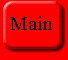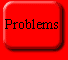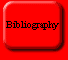## RSA

Before 1974 secure communications was only possible by using private key cryptography. This method implied the following features:

*Anyone who can encipher a message is also able to decipher it

* Sender and receiver share a common secret key which must be transmitted before encryption procedure can start. (usually by some physical means like special couriers, etc. )

Such a private key system is an symmetric system since the same machine (actually private key) can be used to enciphering and deciphering. Yet this is not true for asymmetric systems like public key cryptography.

## What are public-key cryptosystems?

For this kind of secure communications any participant P, has one pair of keys,

* a public key E = Ep and

* a private key D = Dp for deciphering with the property that it is not feasible to compute Dp and Ep.

All public keys are publicly available; they might be stored in a public file. Other hand, the private keys are kept secret; they are known only to their owners.

A public key cryptosystem is called a public key encryption scheme, if for any message m we have

D(E(m)) = m

A public key cryptosystem, is called a public key signature scheme, if for any message m one can verify using the public key E that m and D(m) fit.

Let us now make an example of how two parties can communicate securely over electronic networks.

1. If A wants to send the message m t B, A

* looks up the public key Eb of B.

* enciphers the message m using Eb and

* sends Eb(m) to B.

2. B is able to decipher the ciphertext Eb(m), since he exclusively knows the key Db. :

Db(Eb(m)) = m

3. No other participant can decipher Eb(m), since, by hypothesis, no one can deduce Db from Eb (and Eb(m)).

In order to explain the beauty of public-key crytosystems we will use an everyday analogy.

Suppose that each participant of our imaginary communications system has a mailbox with his name on it, and his own private key. Applying the public key corresponds to inserting a letter to the mailbox. The individuals keys correspond to the secret keys.

If some one wants to send a message to Mrs. Johnson, he simply puts the message into Mrs. Johnson's mailbox. Note how the analogy respects the basic property of a public key system: knowing how to deliver the message does not make the inverse any easier at all.

Now anyone, even the sender may try his key on the mailbox - without any success! Only Mrs. Johnson may open the lock - and she may do so without any difficulty.

* No key exchange among the participants is necessary.

This solves the fundamental problem of private key systems. The important consequence is that public key systems offer spontaneous communications that's MORE SECURE COMMUNICATION! I am in a position to send secret messages to a agent or offshore bank in Cayman Islands without any need to have previously agreed upon a secret key.

* New participants can join the system without any new problems for the old members.

If a new participant joins a symmetric cryptosystem, all three users have to exchange a secret key with him. In this asymmetric system, the old members do not have to update their databases. In short public key systems facilitate secure communications and enable us to COMMUNICATE MORE.

* Public key mechanisms offer an excellent possibility for digital signatures.

How the RSA Algorithm works?

In order to grasp how the RSA algorithm works, we need to understand a theorem proposed by Leonard Euler, a Swiss Mathematician.

Definitions and Assertions for Euler's Theorem

For a natural number n we define O(n) to be the number of positive integers smaller than or equal to n that have no factor except 1 in common with n. In other words, we search for all positive integers smaller than n that are relatively prime to n -- their greatest common divisor with n equals 1. This sounds more complicated than it actually is. Here are some examples:

O(1) = 1, O(2) = 2, O(3) = 2, O(4) = 2,

O(6) = 2, O(10) = 4, O(15) = 8.

We can see the last assertions as follows: The positive integers < 15 that are relatively prime to 15 are 1, 2, 4, 7 ,8, 11, 13, and 14. Since there are 8 different numbers, we have O(15) = 8.

There are also some properties of O(n):

1. If p denotes a prime number, then O(p) = p -1,

because all the p-1 integers 1,2,3,.......,p-1 are relatively prime to p.

2. If p and q are two distinct primes then,

O(pq) = (p-1)(q-1)

This is a particularly important property. There is a total of

pq-1 positive integers smaller than pq. We count how many of them are not relatively prime to pq. On the one hand, these are the q-1 multiples of p, namely

p, 2p, 3p, ........ , (q-1)p,

and on the other hand the p-1 multiples

q, 2q, 2q, 3q, ......, (p-1)q

of q. Since there are the only integers between 1 and pq-1 that are not relatively prime to pq, it follows that

O(pq) = pq -1 - (q-1) -(p-1) = pq-q-p+1 = (p-1)(q-1)

Now we can formulate Euler's Theorem:

Denote by m and n two relatively prime positive integers. Then

mO(n)modn = 1.

We shall be particularly interested in the case, when n is the product of two distinct primes. In the view of the second claim, Euler's Theorem reads as follows:

Let m be an integer that has no common factor except 1 with either of the two distinct primes p and q. Then

m(p-1)(q-1)modpq = 1

In other words, if the large number m(p-1)(q-1) is divided by pq, it leaves 1 as its remainder.

We won't spend time proving this theorem. However we will show a couple of examples:

5O(6)mod6 = 52mod6 = 25 mod 6 =1

can easily be checked. To verify that

31792mod851 = 3122.36mod851

= 31O(23.37)mod851 = 31O(851)851 = 1

would require considerably more effort.

Further Dependents

The RSA algorithm relies on the following facts as well:

* It is extremely difficult to factor a large number.

* Nevertheless, using the Euclidean algorithm it is extremely simple to calculate the gcd of two (even very large numbers.

Computing the GCD:

We shall start with an example. Let a = 792 and b = 75.

792 = 10.75 + 42

75 = 1.42 + 33

42 = 1.33 + 9

33 = 3.9 + 6

9 = 1.6 + 3

Our claim is that 3 is the greatest common divisor of 792 and 75.

Its proof comes from considering the general scheme, as follows:

Eucliedean Algorithm

ro = a

r1 = b

r0 = q1 * r1 + r2 0< r2 <r1

r1 = q2 * r2 + r3 0< r3 < r2

.. ..

ri-1 = qi * ri + ri+1 0< ri+1 < ri

.. ..

rn-2 = qn-1 * rn-2 + rn 0< rn <rn-1

rn-1 = qn * rn.

We claim that the number rn defined by the Euclidean algorithm is the greatest common divisor of a and b. In order to see this, we must demonstrate the following assertions:

* rn divides an and b (that is rn is a common divisor), and

* any number z that divides a and b divides also rn (that is, rn is the greatest among all the common divisors.)

Consider the below equations:

Since rn-1 = qn * rn is a divisor of rn-1.

Hence rn divides qn-1 * rn-1 + rn and hence rn-1.

....

Therefore, rn divides ri (and ri+1) and hence ri-1.

Finally we get that rn divides the numbers r1 (=b) and r0 (=a). Hence, rn is in fact a common divisor of a and b.

We can write the algorithm that does the GCD in a pseudo language:

While b != 0 do

r:= a mod b

a := b, b := r

Write a.

Other Properties With the Modular Inverse

For our purposes the following assertion is of the utmost importance:

Given positive integers a and b with d = gcd(a, b), then there are integers x and y with the property that

d = xa + by.

We will take this for granted, the proof is similar to that of Euclidean Algorithm and not difficult.

This property makes possible another property about relative primes:

Suppose that a and b are integers that are relatively prime (that is, their gcd is 1). Then there is an integer c satisfying

b*c mod a = 1.

This may be restated as: c is the inverse of b modulo a.

We can derive it from the previous result. With that notation we have d = rn = 1. Hence there are integers x and y such that

1 = d = a * x + b * y

Since a * x is a multiple of a, by necessarily has remainder 1 if divided by a. In other words,

b*ymod a = 1.

The assertion follows with c := y.

Here is the summary of all the general properties:

* The Euclidean algorithm may be performed extremely efficiently by a computer.

In particular we note the following:

* It is very easy to compute the gcd of two integers, and, in particular, to decide whether they are relatively prime.

* If a and b are relatively prime, then one can very easily compute an integer c satisfying.

b*c moda =1.

Key Generation:

Before we describe how the RSA algorithm works, we must clarify the hypotheses for the system. First, every participant must get a pair of keys. A key center chooses two distinct large primes p and q and multiples them:

n = pq

Then the center computes

O(n) = O(pq) = (p-1)(q-1)

Finally the center computes two integers d and e with

e*d mod O(n) = 1

The participant gets e and n as his public key and d as his private key.

Certain Remarks:

* No participant P needs to know his secret parameters p, q, and O(n). This ignorance is needed since it is crucial that the other participants not know these numbers. Otherwise they could without any difficulty compute P's private key.

* Are there any security problems with key generation?

There is no problem in computing d and e: the key center first picks an e, either as described in the previous remark, or perhaps by taking any prime not dividing O(n). Another approach would be to choose a random e, compute the gcd of e and O(n) using the Euclidean algorithm, and then divide e by this gcd. Then one would compute a corresponding d using, perhaps, the Euclidean algorithm. Of course, computing O(n) is no problem - of one knows p and q.

Using the RSA Algorithm to Send Messages

If somebody wants to send a message to Mrs. Johnson, he first must learn, Johnson's public key. This public key consists of the modulus n and the exponent e.

Next the message must have the form of one or more positive integers m <= n. There are many ways to achieve this; one method follows. Suppose that the message consists of letters, numbers and special characters. Each character is represented by its own arrangement of eight bits (zeros and ones); most computers use a standard system called ASCII.

So if n has 512 bits, one forms groups of 64 characters each, encodes these characters and gets strings of 64*8 = 512 bits. These bit strings are then interpreted as the binary representations of some numbers m.

We shall assume that our message is a positive integer m<=n. One enciphers such a number m raising it to the eth power and reducing the result modulo n. In thoer words, if

c := memodn,

then c is the ciphertext corresponding to the clear text m.

How does one decipher c? This has been arranged in such a way that only one person can do it, namely the recipient Mrs. Johnson. She simply applies her private key to the cipher text c. More precisely, the number

m'' := cdmodn

is the message Mrs. Johnson gets by deciphering c. This leaves one obvious question. Mrs. Johnson does not care about an arbitrary message m''; she wants to get the original message m. Is m'' = m ?

The following theorem asserts that this is always the case.

Theorem:

For c = memodn. and m'' = cdmodn.

and provided that e*d mod O(n) = 1

For any positive integer m <= n we have m'' = m, in other words the above deciphering algorithm works correctly.

## Proof Of the RSA Algorithm

c^d modn = m^ed mod n
= m^(kO(n)+1) modn for some interger k
= m.m^(kO(n))modn
= mmod n since m^O(n)=1modn
= m since m < n

Is public-key algorithms safe enough?

The question is probably the greatest threat to the RSA algorithm. Is it possible to decipher the message without using the private key?

Suppose we know the public key - that is, the numbers e and

n. How could we compute from this private key d? Naturally, if we knew O(n), then we could compute d; we could proceed as the key generation center and compute O(n) = (p-1)(q-1). Interestingly enough, the converse is also true. If we knew n and O(n), then we could factor n. (Indeed, in this situation we have the two equations

p*q = n and (p-1)(q-1) = O(n)

for the two unknowns p and q. So we could solve for p and q.) To sum up, if n is the product of two distinct primes, then computing O(n) is the same as factoring n.

So everything would be very easy if we could factor n -- and there's the rub. Of course, to the casual eye the obstacle is not immediately obvious; it is a popular belief that since there is no difficulty in factoring integers like 72, 123, or 221, it necessarily follows that factoring larger integers remains relatively easy. But the factorization of even rather small integers like 1763 or 8633 (each a product of a prime) imposes considerable difficulties for a human brain. Considering that the n appearing in the RSA algorithm has a suggested length of about 200 decimal digits, one gets a strong feeling that factoring such a large integers is an extremely difficult problem.

If we wanted to, how could we decompose a larger integer n into its prime factors? A naive approach is to proceed systematically. In other words, one tries every integer m<= n to see whether or not it is a divider of n. Of course, it is not necessary to test all integers <= n. We may restrict ourselves to primes between 2 and sort(n). (For if n = p*q, then p <= sqrt(n) or q <= sqrt(n) )This is a safe procedure, but a very, very lengthy one. For instance, in order to test a 200-digit number, in the worst case, one has to consider all primes between 2 and 10100.

Some Advanced Methods for Public-Key Cryptography

Key Exchange

In view of the fact that today's implementation of public key algorithms are rather slow (while there are good and very fast implementations of symmetric algorithms available), it makes sense to try to combine the two types of algorithms, exploiting each system's advantages. The fundamental idea of a hybrid system is as follows:

Essentially, one uses a symmetric algorithm. A public key encryption system is used only for the exchange of the secret key necessary for the symmetric algorithm. Here the bad performance of the public key algorithm is not a bottleneck for the whole system: the keys to be exchanged are tiny messages (56 or 128 bits) and the actual exchange is relatively infrequent. (A key change for every session is not very often necessary, though occasionally it would be desirable.)

Such a secure key exchange is performed using a public key encryption scheme. In order to send a secret key k to Mr. Whipkey, one must encipher k using Mr. Whipkey's public key. Then Mr. Whipkey deciphers the received value c with this private key and obtains k.

## Shamir's No-key Exchange:

The basic idea is simple . If A wants to encipher a message for B, she first puts the message into a suitcase, secures it by her lock, and sends it to B. He, of course, cannot open A's lock, but he can secure the message also using his key. Then this doubly locked message is sent back to A. Now she may unlock her lock (being no longer able to read the message) and send it again to B. Finally, he removes his lock and can read the message. This procedure is called Shamir's no-key algorithm. For the specific encoding of the mechanism we can use the Massey-Omura scheme.